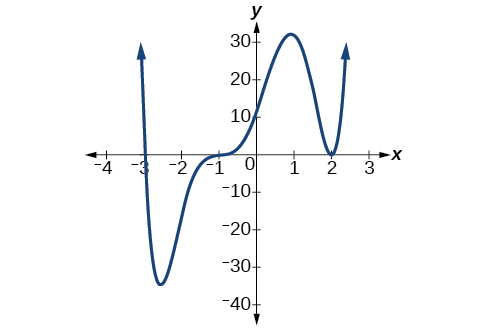# 5.3 Graphs of polynomial functions  (Page 2/13)

 Page 2 / 13

## Finding the x -intercepts of a polynomial function by factoring

Find the x -intercepts of $\text{\hspace{0.17em}}f\left(x\right)={x}^{6}-3{x}^{4}+2{x}^{2}.$

We can attempt to factor this polynomial to find solutions for $\text{\hspace{0.17em}}f\left(x\right)=0.$

$\begin{array}{ccccccccccc}& & & & \hfill \left({x}^{2}-1\right)& =& 0\hfill & & \hfill \left({x}^{2}-2\right)& =& 0\hfill \\ \hfill {x}^{2}& =& 0\hfill & \phantom{\rule{2em}{0ex}}\text{or}& \hfill {x}^{2}& =& 1\hfill & \phantom{\rule{2em}{0ex}}\text{or}& \hfill {x}^{2}& =& 2\hfill \\ \hfill x& =& 0\hfill & & \hfill x& =& ±1\hfill & & \hfill x& =& ±\sqrt{2}\hfill \end{array}$

This gives us five x -intercepts: $\text{\hspace{0.17em}}\left(0,0\right),\left(1,0\right),\left(-1,0\right),\left(\sqrt{2},0\right),\text{\hspace{0.17em}}$ and $\text{\hspace{0.17em}}\left(-\sqrt{2},0\right).\text{\hspace{0.17em}}$ See [link] . We can see that this is an even function because it is symmetric about the y -axis.

## Finding the x -intercepts of a polynomial function by factoring

Find the x -intercepts of $\text{\hspace{0.17em}}f\left(x\right)={x}^{3}-5{x}^{2}-x+5.$

Find solutions for $\text{\hspace{0.17em}}f\left(x\right)=0\text{\hspace{0.17em}}$ by factoring.

$\begin{array}{ccccccccccc}\hfill x+1& =& 0\hfill & \phantom{\rule{2em}{0ex}}\text{or}\phantom{\rule{2em}{0ex}}& \hfill x-1& =& 0\hfill & \phantom{\rule{2em}{0ex}}\text{or}\phantom{\rule{2em}{0ex}}& \hfill x-5& =& 0\hfill \\ \hfill x& =& -1\hfill & & \hfill x& =& 1\hfill & & \hfill x& =& 5\hfill \end{array}$

There are three x -intercepts: $\text{\hspace{0.17em}}\left(-1,0\right),\left(1,0\right),\text{\hspace{0.17em}}$ and $\text{\hspace{0.17em}}\left(5,0\right).\text{\hspace{0.17em}}$ See [link] .

## Finding the y - and x -intercepts of a polynomial in factored form

Find the y - and x -intercepts of $\text{\hspace{0.17em}}g\left(x\right)={\left(x-2\right)}^{2}\left(2x+3\right).$

The y -intercept can be found by evaluating $\text{\hspace{0.17em}}g\left(0\right).$

$\begin{array}{ccc}\hfill g\left(0\right)& =& {\left(0-2\right)}^{2}\left(2\left(0\right)+3\right)\hfill \\ & =& 12\hfill \end{array}$

So the y -intercept is $\text{\hspace{0.17em}}\left(0,12\right).$

The x -intercepts can be found by solving $\text{\hspace{0.17em}}g\left(x\right)=0.$

${\left(x-2\right)}^{2}\left(2x+3\right)=0$
$\begin{array}{ccccccc}\hfill {\left(x-2\right)}^{2}& =& 0\hfill & & \hfill \left(2x+3\right)& =& 0\hfill \\ \hfill x-2& =& 0\hfill & \phantom{\rule{2em}{0ex}}\text{or}\phantom{\rule{2em}{0ex}}& \hfill x& =& -\frac{3}{2}\hfill \\ \hfill x& =& 2\hfill & & & & \end{array}$

So the x -intercepts are $\text{\hspace{0.17em}}\left(2,0\right)\text{\hspace{0.17em}}$ and $\text{\hspace{0.17em}}\left(-\frac{3}{2},0\right).$

## Finding the x -intercepts of a polynomial function using a graph

Find the x -intercepts of $\text{\hspace{0.17em}}h\left(x\right)={x}^{3}+4{x}^{2}+x-6.$

This polynomial is not in factored form, has no common factors, and does not appear to be factorable using techniques previously discussed. Fortunately, we can use technology to find the intercepts. Keep in mind that some values make graphing difficult by hand. In these cases, we can take advantage of graphing utilities.

Looking at the graph of this function, as shown in [link] , it appears that there are x -intercepts at $\text{\hspace{0.17em}}x=-3,-2,\text{\hspace{0.17em}}$ and $\text{\hspace{0.17em}}1.$

We can check whether these are correct by substituting these values for $\text{\hspace{0.17em}}x\text{\hspace{0.17em}}$ and verifying that

$h\left(-3\right)=h\left(-2\right)=h\left(1\right)=0$

Since $\text{\hspace{0.17em}}h\left(x\right)={x}^{3}+4{x}^{2}+x-6,\text{\hspace{0.17em}}$ we have:

$\begin{array}{ccc}\hfill h\left(-3\right)& =& {\left(-3\right)}^{3}+4{\left(-3\right)}^{2}+\left(-3\right)-6=-27+36-3-6=0\hfill \\ \hfill h\left(-2\right)& =& {\left(-2\right)}^{3}+4{\left(-2\right)}^{2}+\left(-2\right)-6=-8+16-2-6=0\hfill \\ \hfill h\left(1\right)& =& {\left(1\right)}^{3}+4{\left(1\right)}^{2}+\left(1\right)-6=1+4+1-6=0\hfill \end{array}$

Each x -intercept corresponds to a zero of the polynomial function and each zero yields a factor, so we can now write the polynomial in factored form.

$\begin{array}{ccc}\hfill h\left(x\right)& =& {x}^{3}+4{x}^{2}+x-6\hfill \\ & =& \left(x+3\right)\left(x+2\right)\left(x-1\right)\hfill \end{array}$

Find the y - and x -intercepts of the function $\text{\hspace{0.17em}}f\left(x\right)={x}^{4}-19{x}^{2}+30x.$

y -intercept $\text{\hspace{0.17em}}\left(0,0\right);\text{\hspace{0.17em}}$ x -intercepts $\text{\hspace{0.17em}}\left(0,0\right),\left(–5,0\right),\left(2,0\right),\text{\hspace{0.17em}}$ and $\text{\hspace{0.17em}}\left(3,0\right)$

## Identifying zeros and their multiplicities

Graphs behave differently at various x -intercepts. Sometimes, the graph will cross over the horizontal axis at an intercept. Other times, the graph will touch the horizontal axis and "bounce" off.

Suppose, for example, we graph the function shown.

$f\left(x\right)=\left(x+3\right){\left(x-2\right)}^{2}{\left(x+1\right)}^{3}$

Notice in [link] that the behavior of the function at each of the x -intercepts is different.Identifying the behavior of the graph at an x -intercept by examining the multiplicity of the zero.

The x -intercept $\text{\hspace{0.17em}}x=-3\text{\hspace{0.17em}}$ is the solution of equation $\text{\hspace{0.17em}}\left(x+3\right)=0.\text{\hspace{0.17em}}$ The graph passes directly through the x -intercept at $\text{\hspace{0.17em}}x=-3.\text{\hspace{0.17em}}$ The factor is linear (has a degree of 1), so the behavior near the intercept is like that of a line—it passes directly through the intercept. We call this a single zero because the zero corresponds to a single factor of the function.

#### Questions & Answers

write down the polynomial function with root 1/3,2,-3 with solution
if A and B are subspaces of V prove that (A+B)/B=A/(A-B)
write down the value of each of the following in surd form a)cos(-65°) b)sin(-180°)c)tan(225°)d)tan(135°)
Prove that (sinA/1-cosA - 1-cosA/sinA) (cosA/1-sinA - 1-sinA/cosA) = 4
what is the answer to dividing negative index
In a triangle ABC prove that. (b+c)cosA+(c+a)cosB+(a+b)cisC=a+b+c.
give me the waec 2019 questions
the polar co-ordinate of the point (-1, -1)
prove the identites sin x ( 1+ tan x )+ cos x ( 1+ cot x )= sec x + cosec x
tanh`(x-iy) =A+iB, find A and B
B=Ai-itan(hx-hiy)
Rukmini
what is the addition of 101011 with 101010
If those numbers are binary, it's 1010101. If they are base 10, it's 202021.
Jack
extra power 4 minus 5 x cube + 7 x square minus 5 x + 1 equal to zero
the gradient function of a curve is 2x+4 and the curve passes through point (1,4) find the equation of the curve
1+cos²A/cos²A=2cosec²A-1
test for convergence the series 1+x/2+2!/9x3ByByByBy Jordon Humphreys